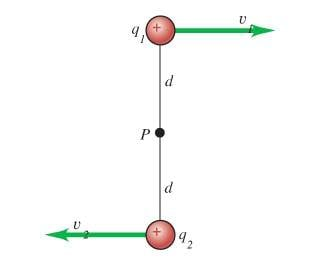# Magnetic Field Intensity and Point Charges

## Homework Statement

Positive point charges q_1= 6.90 microC and q_2= 2.90 microC are moving relative to an observer at point P as shown in the figure. The distance from the observer to either charge is originally d = 0.190 m.The two charges are at the locations shown in the figure. Charge q_1 is moving at a speed of v_1 = 3.20×10^6 m/s and q_2 is moving at a speed of v_2 = 9.40×10^6 m/s.

a. What is the magnitude of the net magnetic field they produce at point P ?
b. What is the direction of the net magnetic field they produce at point P ?See below.

## The Attempt at a Solution

I think I mixed up my signs for the cross product of v and r. Are the two fields in opposite directions? Any advice on those parts are appreciated.

B_1 = (mu_0/4*pi)*[(q*v vector*r vector)/(r^3)]

v vector = 3.20*10^6 m/s*i
r vector = (-0.190 m)*j

v vector*r vector = (3.20*10^6 m/s)i*(-0.190 m)j = -60800 m^2/s*k

B_1 = [(1*10^-7 T*m/A)*(6.90*10^-6 C)(-60800 m^2/s*k)]/[0.190 m]^3

= (-6.12*10^-5 T)*k ??

B_2 = (mu_0/4*pi)*[(q*v vector*r vector)/(r^3)]

v vector = -9.40*10^6 m/s*i
r = 0.190 m*j

v vector*r vector = (-9.40*10^6 m/s*i)*(0.190m*j) = -1786000 m^2/s

B_2 = [(1*10^-7 T*m/A)*(2.90*10^-6 C)(-1786000 m^2/s*k)]/[0.190 m]^3
= (-7.55*0^-5 T)*k ??

B_total = (-6.12*10^-5 T)*k - (-7.55*0^-5 T)*k = -(1.37*10^-4 T)*k out of the page?? (negative z-axis?)

Thanks.